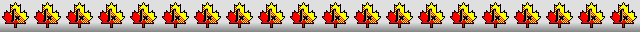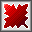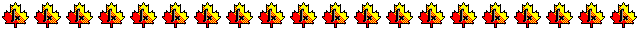Maple worksheets on the derivation of order 7 Runge-Kutta schemesOrder conditions for an order 7 Runge-Kutta scheme - RKcoeff7a.mws

• Order conditions for an order 7 Runge-Kutta scheme and their associated rooted trees.

Derivation of 10 stage, combined order 6 and 7 Runge-Kutta schemes - RKcoeff7b.mws

Derivation of 11 stage, order 7 Runge-Kutta schemes, each with a 12 stage order 6 FSAL embedded scheme - RKcoeff7c.mws

Derivation of 10 stage, order 7 Runge-Kutta schemes, each with an embedded order 6 scheme - RKcoeff7d.mws

• Some schemes due to Tanaka, Muramatsu and Yamashita together with similar schemes.
See: On the Optimization of Some Nine-Stage Seventh-order Runge-Kutta Method, by M. Tanaka, S. Muramatsu and S. Yamashita,
Information Processing Society of Japan, Vol. 33, No. 12 (1992) pages 1512-1526.

• Coefficients for the schemes and some properties
RKcoeff7d_1.pdf     RKcoeff7d_2.pdf     RKcoeff7d_3.pdf     RKcoeff7d_4.pdf

Derivation of 11 stage, combined order 6 and 7 Runge-Kutta schemes- RKcoeff7e.mws

• A scheme due to P. Sharp and E. Smart together with similar schemes.
See: Explicit Runge-Kutta Pairs with One More Derivative Evaluation than the Minimum, by P.W.Sharp and E.Smart,
Siam Journal of Scientific Computing, Vol. 14, No. 2, pages. 338-348, March 1993.

• Coefficients for the schemes and some properties
RKcoeff7e_1.pdf     RKcoeff7e_2.pdf     RKcoeff7e_3.pdf     RKcoeff7e_4.pdf     RKcoeff7e_5.pdf

Procedures related to the construction of Runge-Kutta schemes - butcher.zip

Procedures for root-finding and determining minimum points  - roots.zip

Procedures for numerical integration  - intg.zip

Top of page

Main index